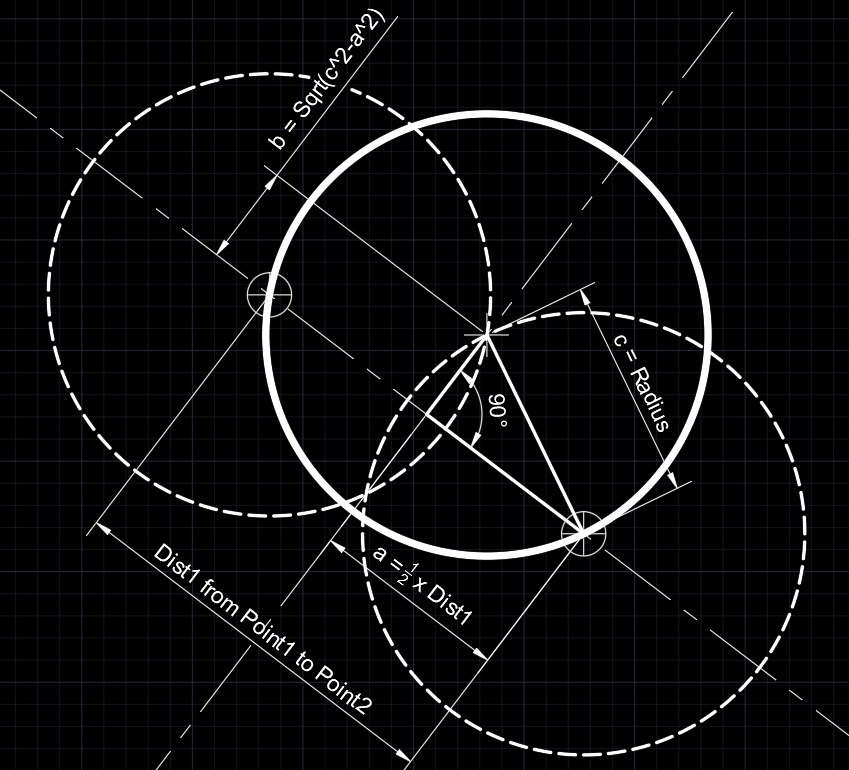AutoCAD has a feature to draw circle with a Tangent, Tangent and Radius; however, this is not the same. This is a AutoLisp program to draw a Circle from two given points along the circumference of a circle with a given radius.

Years ago when drafting by hand, you would use a compass with the desired radius and draw two arcs from each given point to determine the correct origin of the desired circle.

Two circles have two intersection points, when using CPPR in AutoCAD select the two points in a clockwise direction will gives a circle in a predictable place.

(defun c:cppr ()
(setq Point1 (getpoint "First Point: "))
(setq Point2 (getpoint Point1 "Second Point: "))
(setq Dist1 (distance Point1 Point2))
(setq Ang1 (angle Point1 Point2))